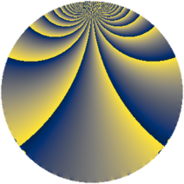# Properties

 Label 690.2.jLevel $690$ Weight $2$ Character orbit 690.j Rep. character $\chi_{690}(367,\cdot)$ Character field $\Q(\zeta_{4})$ Dimension $48$ Newform subspaces $2$ Sturm bound $288$ Trace bound $6$

# Related objects

## Defining parameters

 Level: $$N$$ $$=$$ $$690 = 2 \cdot 3 \cdot 5 \cdot 23$$ Weight: $$k$$ $$=$$ $$2$$ Character orbit: $$[\chi]$$ $$=$$ 690.j (of order $$4$$ and degree $$2$$) Character conductor: $$\operatorname{cond}(\chi)$$ $$=$$ $$115$$ Character field: $$\Q(i)$$ Newform subspaces: $$2$$ Sturm bound: $$288$$ Trace bound: $$6$$ Distinguishing $$T_p$$: $$7$$

## Dimensions

The following table gives the dimensions of various subspaces of $$M_{2}(690, [\chi])$$.

Total New Old
Modular forms 304 48 256
Cusp forms 272 48 224
Eisenstein series 32 0 32

## Trace form

 $$48 q + O(q^{10})$$ $$48 q + 16 q^{13} - 48 q^{16} + 8 q^{23} - 32 q^{25} - 16 q^{26} + 32 q^{31} - 16 q^{35} + 48 q^{36} + 32 q^{47} + 16 q^{50} + 16 q^{52} - 32 q^{55} - 64 q^{62} + 48 q^{71} - 64 q^{73} + 32 q^{75} + 16 q^{77} + 16 q^{78} - 48 q^{81} + 48 q^{82} - 48 q^{85} + 32 q^{87} + 8 q^{92} + 48 q^{93} + 48 q^{95} - 32 q^{98} + O(q^{100})$$

## Decomposition of $$S_{2}^{\mathrm{new}}(690, [\chi])$$ into newform subspaces

Label Dim $A$ Field CM Traces $q$-expansion
$a_{2}$ $a_{3}$ $a_{5}$ $a_{7}$
690.2.j.a $24$ $5.510$ None $$0$$ $$0$$ $$0$$ $$0$$
690.2.j.b $24$ $5.510$ None $$0$$ $$0$$ $$0$$ $$0$$

## Decomposition of $$S_{2}^{\mathrm{old}}(690, [\chi])$$ into lower level spaces

$$S_{2}^{\mathrm{old}}(690, [\chi]) \cong$$ $$S_{2}^{\mathrm{new}}(115, [\chi])$$$$^{\oplus 4}$$$$\oplus$$$$S_{2}^{\mathrm{new}}(230, [\chi])$$$$^{\oplus 2}$$$$\oplus$$$$S_{2}^{\mathrm{new}}(345, [\chi])$$$$^{\oplus 2}$$